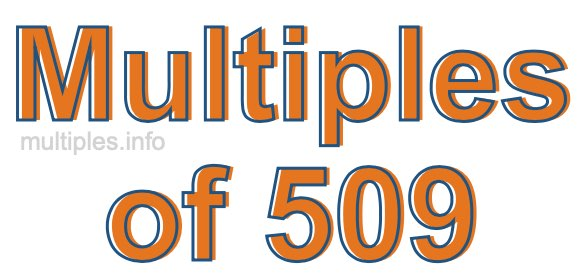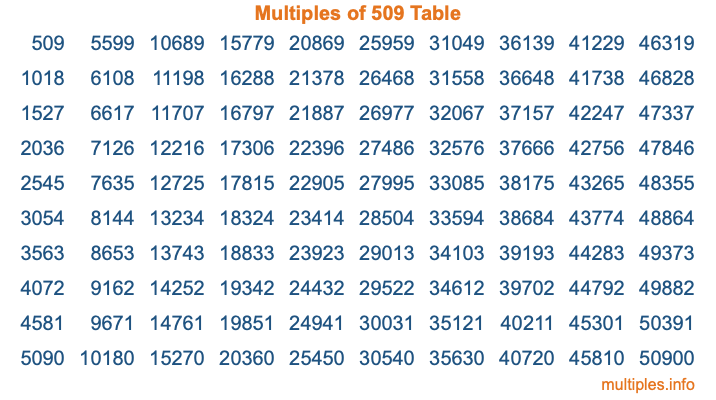Multiples of 509Welcome to the Multiples of 509 page. Here we will first teach you everything you will ever need to know about the multiples of 509, and then give you a study guide summary of everything we taught you to make sure you remember it all. Use this page to look up facts and learn information about the multiples of 509. This page will make you a multiples of five hundred nine expert!

Definition of Multiples of 509
Multiples of 509 are all the numbers that when divided by 509 equal an integer. Each of the multiples of 509 are called a multiple. A multiple of 509 is created by multiplying 509 by an integer.

Therefore, to create a list of multiples of 509, you start with 1 multiplied by 509, then 2 multiplied by 509, then 3 multiplied by 509, and so on for as long as you want. Thus, the list of the first five multiples of 509 is 509, 1018, 1527, 2036, and 2545. To see a larger list of multiples of 509, see the printable image of Multiples of 509 further down on this page. We also have a category where you can choose any nth multiple of 509.

Multiples of 509 Checker
The Multiples of 509 Checker below checks to see if any number of your choice is a multiple of 509. In other words, it checks to see if there is any number (integer) that when multiplied by 509 will equal your number. To do that, we divide your number by 509. If the the quotient is an integer, then your number is a multiple of 509.

Is  a multiple of 509?

Least Common Multiple of 509 and ...
A Least Common Multiple (LCM) is the lowest multiple that two or more numbers have in common. This is also called the smallest common multiple or lowest common multiple and is useful to know when you are adding our subtracting fractions. Enter one or more numbers below (509 is already entered) to find the LCM.

Check out our LCM Calculator if you need more details about the Least Common Multiple or if you need the LCM for different numbers for adding and subtraction fractions.

nth Multiple of 509
As we stated above, 509 is the first multiple of 509, 1018 is the second multiple of 509, 1527 is the third multiple of 509, and so on. Enter a number below to find the nth multiple of 509.

th multiple of 509

Multiples of 509 vs Factors of 509
509 is a multiple of 509 and a factor of 509, but that is where the similarities end. All postive multiples of 509 are 509 or greater than 509. All positive factors of 509 are 509 or less than 509.

Below is the beginning list of multiples of 509 and the factors of 509 so you can compare:

Multiples of 509: 509, 1018, 1527, 2036, 2545, etc.

Factors of 509: 1, 509

As you can see, the multiples of 509 are all the numbers that you can divide by 509 to get a whole number. The factors of 509, on the other hand, are all the whole numbers that you can multiply by another whole number to get 509.

It's also interesting to note that if a number (x) is a factor of 509, then 509 will also be a multiple of that number (x).

Multiples of 509 vs Divisors of 509
The divisors of 509 are all the integers that 509 can be divided by evenly. Below is a list of the divisors of 509.

Divisors of 509: 1, 509

The interesting thing to note here is that if you take any multiple of 509 and divide it by a divisor of 509, you will see that the quotient is an integer.

Multiples of 509 Table
Below is an image of the first 100 multiples of 509 in a table. The table is in chronological order, column by column. The first column has the first ten multiples of 509, the second column has the next ten multiples of 509, and so on.The Multiples of 509 Table is also referred to as the 509 Times Table or Times Table of 509. You are welcome to print out our table for your studies.

Negative Multiples of 509
Although not often discussed or needed in math, it is worth mentioning that you can make a list of negative multiples of 509 by multiplying 509 by -1, then by -2, then by -3, and so on, to get the following list of negative multiples of 509:

-509, -1018, -1527, -2036, -2545, etc.

Multiples of 509 Summary
Below is a summary of important Multiples of 509 facts that we have discussed on this page. To retain the knowledge on this page, we recommend that you read through the summary and explain to yourself or a study partner why they hold true.

There are an infinite number of multiples of 509.

A multiple of 509 divided by 509 will equal a whole number.

509 divided by a factor of 509 equals a divisor of 509.

The nth multiple of 509 is n times 509.

The largest factor of 509 is equal to the first positive multiple of 509.

509 is a multiple of every factor of 509.

509 is a multiple of 509.

A multiple of 509 divided by a divisor of 509 equals an integer.

509 divided by a divisor of 509 equals a factor of 509.

Any integer times 509 will equal a multiple of 509.

Multiples of a Number
Here you can get the multiples of another number, all with the same attention to detail as we did for multiples of 509 on this page.

Multiples of
Multiples of 510
Did you find our page about multiples of five hundred nine educational? Do you want more knowledge? Check out the multiples of the next number on our list!